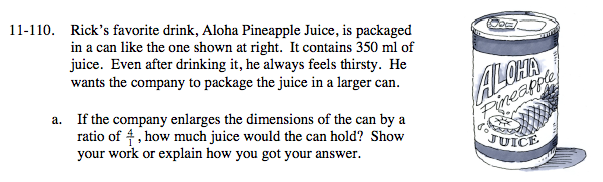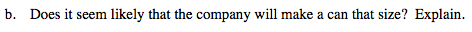### Home > MC2 > Chapter 11 > Lesson 11.3.3 > Problem11-110

11-110.$\text{If the scale factor is \frac{4}{1}, the volume factor is (\frac{4}{1})^3}$

If the original volume is 350 ml and the scale factor
is 64 times as large, what is the new volume?

350 ml · 64 = 22,400 ml22 liters would weight almost 50 pounds!